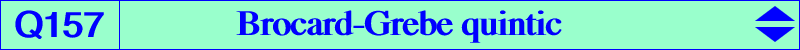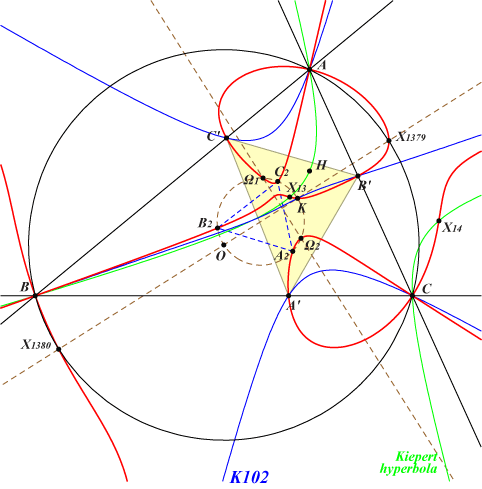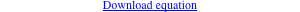∑ a^2 y^2 z^2 [2 (b^2 - c^2) SA x + a^2 (c^2 y - b^2 z)] = 0X(6), X(13), X(14), X(1379), X(1380), X(39641), X(39642) isogonal conjugate of X(10221) infinite points of K102 Ω1, Ω2 : Brocard points A2, B2, C2 : vertices of the second Brocard triangle A', B', C' : vertices of the cevian triangle of X(6) other points belowQ157 is an example of curve that passes through the Rescassol-Skoubidou points mentioned in page K003. The isogonal transform of Q157 is the circular quartic Q158. Q157 is a circular quintic with three real asymptotes parallel to those of the Grebe cubic K102 and two imaginary asymptotes passing through X(182), the center of the Brocard circle. A, B, C are three nodes on the curve with nodal tangents the bisectors of ABC. It follows that Q157 meets these six bisectors at six pairs of points, each pair on one bisector. These 12 points form 3 groups of 4 points and 4 points lie on a rectangular circum-hyperbola whose center is a midpoint of ABC. These hyperbolas are the isogonal transforms of the perpendicular bisectors of ABC. Q157 meets the Brocard axis at X(6), X(1379), X(1380) and the imaginary foci X(39641), X(39642) of the Brocard ellipse. These latter points lie on the Kiepert hyperbola and on K003. Q157 meets the focal axis X(39)X(512) of the Brocard ellipse at the Brocard points Ω1, Ω2 and three (not always all real) other points which lie on the three cubics pK(X9468, X6), pK(X14251, X694), pK(X694^2, X694^2 ÷ X6). These cubics are in a same pencil which contains the union of the focal axis and the circum-conic passing through G and K. Q157 meets the Fermat axis at X(6), X(13), X(14) and two other points on the circum-conic with perspector X(50), passing through X(54), X(110). This is the isogonal transform of the perpendicular bisector of OH. *** A related cubic The locus of P whose polar conic in Q157 is a rectangular hyperbola (i.e. the Laplacian of Q157) is a circum-cubic (K) with three asymptotes concuring at X(40108), the complement of X(7697). These asymptotes are parallel to those of pK(X6, X576). This cubic (K) meets (O) at the same points as pK(X6, X40107), where X(40107) is the complement of X(576). Recall that the polar conics of A, B, C are decomposed into the pairs of bisectors of ABC.